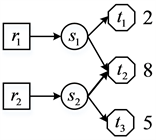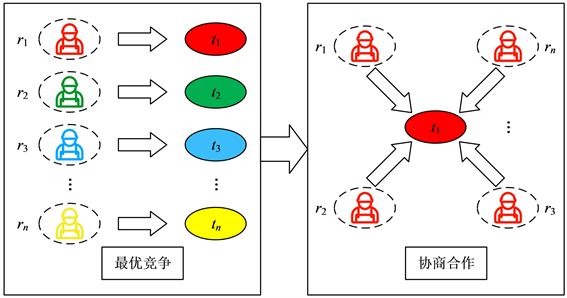#### 期刊菜单

Two-Stage Multi-Agent Task Allocation Algorithm Considering Market Weight
DOI: 10.12677/HJDM.2022.122017, PDF , HTML, XML, 下载: 208  浏览: 397

Abstract: At present, the hot issues in multi-agent system are multi-agent resource allocation and multi-agent task allocation, that is, how to allocate appropriate tasks to appropriate agents to optimize the overall task allocation results. However, considering the self-interest of the agent, there is little re-search on the problem. In order to effectively solve the problem of task allocation of self-interest agent, a two-stage task allocation model including optimal competition and market cooperation is constructed. On the basis of optimal competition, the market cooperation stage further coordinates and optimizes the optimal task allocation. Considering that the relationship between candidates may ultimately affect the overall income of the market, the concept of “market weight” is proposed to describe a more accurate cooperative relationship. The two-stage allocation model based on market weight can not only enable task candidates to give full play to their own advantages, participate in competition and protect the interests of task candidates, but also optimize the overall in-come of the market. Finally, the simulation results verify the effectiveness of the proposed algorithm.

1. 引言

2. 相关工作

3. 问题模型定义

$T:=\left\{{t}_{1},{t}_{2},\cdots ,{t}_{m}\right\}$，其中 $n=|R|,l=|S|,m=|T|$。其中 $|\ast |$ 代表每一个集合中所包含元素的个数。当 $i=1,2,\cdots ,n$$j=1,2,\cdots ,l$$k=1,2,\cdots ,m$$R{S}_{i,j}=1$ 表示用户 ${r}_{i}$ 拥有技能 ${s}_{j}$$S{T}_{j,k}=1$ 完成任务 ${t}_{k}$ 需要技能 ${s}_{j}$，否则不需要，任务相应的效益使用向量 $U:\left\{{u}_{1},{u}_{2},\cdots ,{u}_{m}\right\}$ 表示。TS表示所有任务的效益分配方案，当 $k=1,2,\cdots ,m$$j=1,2,\cdots ,l$$T{S}_{k,\tau }\left(\tau \right)$ 表示在 $\tau$ 时刻， ${t}_{k}$ 分配给 ${s}_{j}$ 的收益份额。如果 $S{T}_{i,j}=0$，则 $T{S}_{k,j}\left(\tau \right)=-2$

${r}_{i}\left(\tau \right):=〈R{S}_{i,\cdot },cos{t}_{i,\cdot },{w}_{i}\left(\tau \right),R{T}_{i,\cdot }\left(\tau \right),provid{e}_{i,\cdot }\left(\tau \right),r{u}_{i,\cdot }\left(\tau \right),RInsy{s}_{i}\left(\tau \right)〉$，矩阵RS的第i行用 $R{S}_{i,\cdot }$ 表示，代表了用户 ${r}_{i}$ 拥有的技能。 $cos{t}_{i,\cdot }$ 为矩阵 $cost$ 的第i行，表示用户 ${r}_{i}$ 使用相应技能所需花费的成本。 ${w}_{i}\left(\tau \right)$ 表示在 $\tau$ 时刻该用户 ${r}_{i}$ 的市场权重。 $R{T}_{i,\cdot }\left(\tau \right)$$RT\left(\tau \right)$ 矩阵的第i行，代表用户 ${r}_{i}$ 的当前任务选择情况。 $provid{e}_{i,\cdot }\left(\tau \right)$ 代表技能分配矩阵 $provide\left(\tau \right)$ 第i行， $provid{e}_{i,\cdot }\left(\tau \right)$ 是一个包含l个元素的向量， $provid{e}_{i,j}\left(\tau \right)=1$ 代表用户 ${r}_{i}$ 为当前选择的任务提供了技能 ${s}_{j}$，否则不为其提供。 $r{u}_{i,\cdot }\left(\tau \right)$ 为一个包含m个元素的向量， $r{u}_{i}\left(\tau \right):=\left\{r{u}_{i,1}\left(\tau \right),\cdots ,r{u}_{i,k}\left(\tau \right),\cdots ,r{u}_{i,m}\left(\tau \right)\right\}$，其中 $r{u}_{i,k}\left(\tau \right)\ge 0\text{\hspace{0.17em}}\left(k\in \left\{1,2,\cdots ,m\right\}\right)$ 代表如果用户 ${r}_{i}$ 在时刻 $\tau$ 选择任务m将会获得的收益。 $RInsy{s}_{i}\left(\tau \right)$ 表示当前用户在系统中的存在状态， $RInsy{s}_{i}\left(\tau \right)=1$ ( $RInsy{s}_{i}\left(\tau \right)=0$)代表当前用户已经选定一个能够完成的任务并且退出市场(在系统中待选择任务)。

${t}_{k}\left(\tau \right):=〈S{T}_{\cdot ,k},TS{N}_{k},{u}_{k},R{T}_{\cdot ,k}\left(\tau \right),{T}_{k}InSys\left(\tau \right)〉$。其中 $S{T}_{\cdot ,k}$ 代表ST的的第k列，是一个包含l个元素的列向

$R{T}_{\cdot ,k}\left(\tau \right)$ 代表任务分配矩阵 $RT\left(\tau \right)$ 的第k列，代表当前任务被哪些用户选择。 ${T}_{k}Insy{s}_{i}\left(\tau \right)=1$ 代表在时刻 $\tau$ 任务已经获得所有的技能，并且能被完成，下一步将该任务从市场中剔除。 ${T}_{k}Insy{s}_{i}\left(\tau \right)=-1$ 代表在时刻 $\tau$，任务 ${t}_{k}$ 没有找到全部所需技能或者完成该任务 ${t}_{k}$ 所需的用户的技能成本大于所能提供的收益，则将该任务从市场中删除。 ${T}_{k}Insy{s}_{i}\left(\tau \right)=0$ 表示在时刻 $\tau$，任务 ${t}_{k}$ 处于待完成状态。

$R{T}_{ij}=\left\{\begin{array}{cc}1& {r}_{i}\text{ }\text{ }选择任务\text{ }\text{ }{t}_{j}\\ 0& {r}_{i}\text{ }\text{ }不选择任务\text{ }\text{ }{t}_{j}\end{array}$

$\left\{\begin{array}{l}Sum=\left[\begin{array}{ccc}su{m}_{11}& \cdots & su{m}_{1k}\\ ⋮& \ddots & ⋮\\ su{m}_{n1}& \cdots & su{m}_{nk}\end{array}\right]\\ Cost=\left[\begin{array}{ccc}cos{t}_{11}& \cdots & cos{t}_{1k}\\ ⋮& \ddots & ⋮\\ cos{t}_{n1}& \cdots & cos{t}_{nk}\end{array}\right]\end{array}$

$su{m}_{ij}$ 代表任务 ${t}_{j}$ 分配给用户agent ${r}_{i}$ 的份额。 $cos{t}_{ij}$ 代表用户agent ${r}_{i}$ 完成任务 ${t}_{j}$ 需要付出的技能成本。

$\left\{\begin{array}{l}provide\left[\begin{array}{ccc}provid{e}_{11}& \cdots & provid{e}_{1j}\\ ⋮& \ddots & ⋮\\ provid{e}_{k1}& \cdots & provid{e}_{kj}\end{array}\right]\\ revenue\left[\begin{array}{ccc}revenu{e}_{11}& \cdots & revenu{e}_{1j}\\ ⋮& \ddots & ⋮\\ revenu{e}_{k1}& ⋮& revenu{e}_{kj}\end{array}\right]\end{array}$

$\left\{\begin{array}{l}SumRT=\underset{i=1}{\overset{n}{\sum }}\underset{j=1}{\overset{k}{\sum }}su{m}_{ij}R{T}_{ij}\\ SumCost=\underset{i=1}{\overset{n}{\sum }}\underset{j=1}{\overset{k}{\sum }}su{m}_{ij}cos{t}_{ij}\end{array}$

$SysRev\left(\tau \right)=SumRT\left(\tau \right)-SumCost\left( \tau \right)$

4. 基于市场协作的多agent任务分配模型

4.1. 阶段1的任务选择策略

(1) $P\left(i,k,\tau \right)$ 代表在时刻 $\tau$，用户 ${r}_{i}$ 能为任务 ${t}_{k}$ 提供的技能的集合，记为 ${P}_{num}\left(i,k,\tau \right)$，其计算公式如下所示：

${p}_{num}\left(i,k,\tau \right):=\left\{\begin{array}{l}{P}_{num}\left(i,k,\tau -1\right)+left\left(\tau \right),R{T}_{i,k}\left(\tau -1\right);\\ nee{d}_{i,k}-\underset{j=1}{\overset{l}{\sum }}{I}_{2}\left(i,j,k,\tau \right),否则\end{array}$

${p}_{num}\left(i,k,\tau \right)=0$，则代表任务 ${t}_{k}$ 所需的技能不能被用户 ${r}_{i}$ 提供。 $\underset{i\text{'}\in \left\{1,\cdots ,n\right\},i\text{'}\ne i}{\sum }R{T}_{i\text{'},k}\left(\tau \right)\cdot provid{e}_{i\text{'},j}\left(\tau \right)=0$ 代表当

$\underset{i\text{'}\in \left\{1,\cdots ,n\right\},i\text{'}\ne i}{\sum }R{T}_{i\text{'},k}\left(\tau -n\right)\cdot provid{e}_{i\text{'},j}\left(\tau -n\right)\ge 1$ 则代表了用户 ${r}_{i}$$\tau$ 时刻选择任务时，任务 ${t}_{k}$ 所需技能 ${s}_{j}$ 已被

$R/\left\{{r}_{i}\right\}$ 中的一个用户提供。

(2) ${N}_{i}\left(\tau \right):=\left\{{t}_{k}\in T|P\left(i,k,\tau \right)\ne \varnothing \right\}$

(3) $complete\left(i,k,\tau \right)$ 代表如果用户 ${r}_{i}$ 在时刻 $\tau$ 选择任务k，任务k能不能完成。

$complete\left(i,k,\tau \right):=\left\{\begin{array}{l}1,R{T}_{i,k}\left(\tau \right)=1\wedge \underset{j=1}{\overset{l}{\sum }}{I}_{1}\left(i,k,\tau \right)=TS{N}_{k}\\ 0,否则\end{array}$

$RT\left(\tau \right)$ 的任务分配情境下，若任务 ${t}_{k}$ 拥有所需的技能 ${s}_{j}$，则 ${I}_{1}\left(j,k,\tau \right)=1$，任务 ${t}_{k}$ 没有获得所需技能 ${s}_{j}$ 或所需技能在市场中所有用户 ${r}_{i}$ 都无法提供时，则 ${I}_{2}\left(j,k,\tau \right)=0$

(4) ${C}_{i}\left(\tau \right):=\left\{{t}_{k}\in T|complete\left(i,k,\tau \right)=1\right\}$

(5) $r{u}_{i,k}\left(\tau \right)$ 代表用户 ${r}_{i}$ 在时刻 $\tau$ 选择任务 ${t}_{k}$，则用户 ${r}_{i}$ 能获得个体收益，其计算公式如下：

$r{u}_{i,k}\left(\tau \right)=\left\{\begin{array}{l}\frac{{u}_{k}}{TS{N}_{k}}\cdot {p}_{num}\left(i,k,\tau \right),\text{}complete\left({t}_{k},i,\tau \right)\wedge {p}_{num}\left(i,k,\tau \right)\ne 1\\ 0,\text{}否则\end{array}$

4.2. 下面简单说明算法第一阶段的收敛性

4.3. 阶段二的任务选择策略

4.4. 简单说明阶段二算法的有效性

5. 实例分析Figure 1. Graphic representation of coalitional skill game modelFigure 2. The shift in Market conditions

6. 仿真结果

6.1. 普通遗传算法最优参数的选取

6.2. 算法性能比较Table 1. The average system returns of common genetic algorithmsTable 2. The system revenue and uptime under randomly generated dataset 2Table 3. The system revenue and uptime under randomly generated dataset 3Table 4. The system revenue and runtime based on the data set 3 generated by iMPOSETable 5. The system revenue and runtime based on the data set 4 generated by iMPOSE

7. 总结

NOTES

*第一作者。

#通讯作者。

  Sycara, P.K. (1998) Muti-Agent Systems. AI Magazine, 19, 79-92.  Jennings, N.R. (1995) Controlling Cooperative Problem Solving in Industrial Multi-Agent Systems Using Joint Intentions. Artificial Intelligence, 75, 195-240. https://doi.org/10.1016/0004-3702(94)00020-2  Kraus, S. and Arkin, R.C. (2001) Strategic Negotiation in Multiagent Environments. MIT Press, Cambrigde.  陈琦. 2020年9月新能源车销售三强:上汽通用五菱、比亚迪、特斯拉[J]. 汽车与配件, 2020(20): 43.  Bachrach, Y., Parkes, D.C. and Rosenschein, J.S. (2013) Computing Co-operative Solution Concepts in Coalitional Skill Games. Artificial Intelligence, 204, 1-21. https://doi.org/10.1016/j.artint.2013.07.005  蒋建国, 苏兆品, 张国富, 夏娜. 多任务联盟形成中的Agent行为策略研究[J]. 控制理论与应用, 2008, 25(5): 853-856.  Cisneros-Cabrera, S., Sampaio, P. and Mehandjiev, N. (2018) A B2B Team Formation Microservice for Collaborative Manufacturing in Industry 4.0. 2018 IEEE World Congress on Services (SERVICES), San Francisco, 2-7 July 2018, 37-38. https://doi.org/10.1109/SERVICES.2018.00032  Hayano, M., Hamada, D. and Sugawara, T. (2014) Role and Member Selection in Team Formation Using Resource Estimation for Large-Scale Multi-Agent Systems. Neurocompu-ting, 146, 164-172. https://doi.org/10.1016/j.neucom.2014.04.059  Juárez, J. and Brizuela, C.A. (2018) A Multi-Objective For-mulation of the Team Formation Problem in Social Networks: Preliminary Results. Proceedings of the Genetic and Evo-lutionary Computation Conference, Kyoto, 15-19 July 2018, 261-268. https://doi.org/10.1145/3205455.3205634  Zzkarian, A. and Kusiak, A. (1999) Forming Teams: An Analytical Approach. IIE Transactions, 31, 85-97. https://doi.org/10.1080/07408179908969808  Kara, I. and Bektas, T. (2006) Integer Linear Programming Formulations of Multiple Salesman Problems and Its Variations. European Journal of Operational Research, 174, 1449-1458. https://doi.org/10.1016/j.ejor.2005.03.008  Wooldridge, M. and Dunne, P.E. (2004) On the Com-putational Complexity of Qualitative Coalitional Games. Artificial Intelligence, 158, 27-73. https://doi.org/10.1016/j.artint.2004.04.002  Wooldridge, M. and Dunne, P.E. (2006) On the Computational Complexity of Coalitional Resource Games. Journal of Artificial Intelligence, 170, 835-871. https://doi.org/10.1016/j.artint.2006.03.003  张国富, 蒋建国, 夏娜, 苏兆品. 基于离散粒子群算法求解复杂联盟生成问题[J]. 电子学报, 2007, 35(2): 323-327.  Larson, K.S. and Sandholm, T.W. (2000) Anytime Coali-tion Structure Generation: An Average Case Study. Journal of Experimental & Theoretical Artificial Intelligence, 12, 23-42. https://doi.org/10.1080/095281300146290  苏射雄, 胡山立, 郑盛福, 林超峰, 骆剑彬. 基于势结构的任一时间联盟结构生成算法[J]. 计算机研究与发展, 2008, 45(10): 1756-1762.  梁军, 程显毅. 基于混合蚁群遗传算法的agent联盟求解[J]. 计算机科学, 2009, 36(5): 227-231.  桂海霞, 张国富, 苏兆品, 蒋建国. 一种基于差分进化和编码修正的重叠联盟结构生成算法[J]. 控制理论与应用, 2018, 35(2): 215-223.  许波, 余建平. 基于QPSO的单任务Agent联盟形成[J]. 计算机工程, 2010, 36(19): 168-170.  尹蕾. 分布式智能系统中多任务协作机制研究[D]: [博士学位论文]. 合肥: 合肥工业大学, 2019.  Vig, L. and Adams, J.A. (2006) Multi-Robot Coalition Formation. IEEE Transactions on Robotics, 22, 637-649. https://doi.org/10.1109/TRO.2006.878948  Service T.C. and Adams J.A. (2011) Coalition Formation for Task Allocation: Theory and Algorithms. Autonomous Agents and Multi-Agent Systems, 22, 225-248. https://doi.org/10.1007/s10458-010-9123-8  Lin, L. and Zheng, Z. (2005) Combinatorial Bids Based Mul-ti-Robot Task Allocation Method. Proceedings of the 2005 IEEE International Conference on Robotics and Automation, Barcelona, 18-22 April 2005, 1145-1150. https://doi.org/10.1109/ROBOT.2005.1570270  Sisikoglu, E., Epelman, M.A. and Smith, R.L. (2011) A Sampled Fictitious Play Based Learning Algorithm for Infinite Horizon Markov Decision Processes. Proceedings of the 2011 Winter Simulation Conference, Phoenix, 11-14 December 2011, 4086-4097. https://doi.org/10.1109/WSC.2011.6148098  Myszkowski, P.B., Skowroński, M.E. and Sikora, K. (2015) A New Benchmark Dataset for Multi-Skill Resource- Constrained Project Scheduling Problem. Proceedings of the 2015 Federated Conference on Computer Science and Information Systems (FedCSIS), Łódź, 13-16 September 2015, 129-138. https://doi.org/10.15439/2015F273  Myszkowski, P.B., Skowroński, M.E., Olech, Ł.P., et al. (2015) Hybrid Ant Colony Optimization in Solving Multi-Skill Resource-Constrained Project Scheduling Problem. Soft Computing, 19, 3599-3619. https://doi.org/10.1007/s00500-014-1455-x